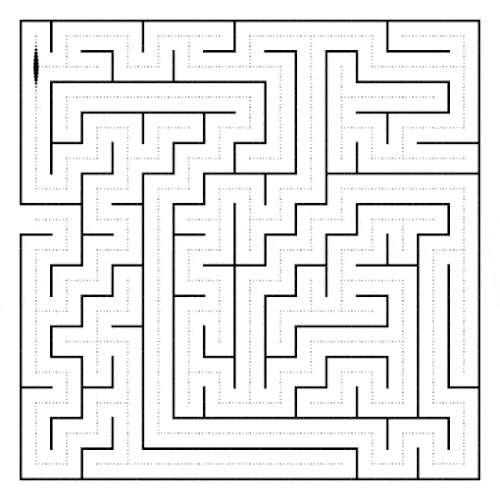``````// 其中 choices 表示我们能做出的选择，selected 表示已选择的路径
void backtracking(vecotr<int> choices, vector<int> selected) {

if (end) return;

for choice in choices {
// 进行选择
selected.push_back(choice);

// 继续前行
backtracking(choices, selected);

// 撤销选择
selectd.pop_back();
}
}
``````

``````给定一个没有重复数字的序列，返回其所有可能的全排列

Example:
Input: nums = [1,2,3]
Output: [[1,2,3],[1,3,2],[2,1,3],[2,3,1],[3,1,2],[3,2,1]]
``````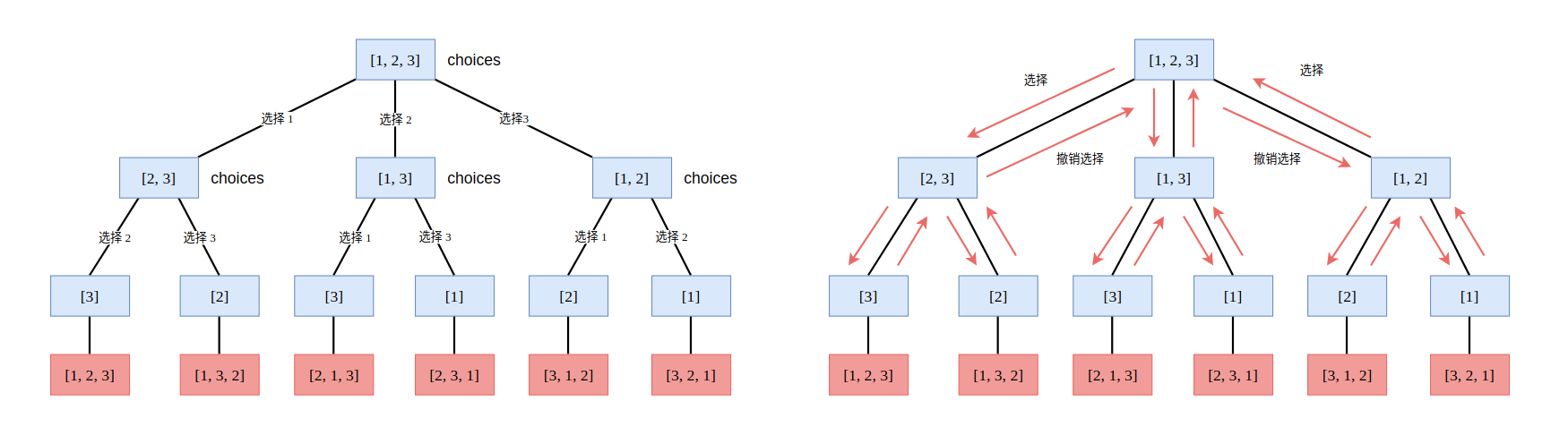``````class Solution {

// 保存每一个排列结果
vector<vector<int>> result;

// 在每次的选择中，我们不能够再选择哪些已经选择过的元素了
// 所以我们使用一个数组来记录已经选择过的元素索引
// visted[i] = true 则表示第 i 个元素已经被选择
vector<bool> visted;

// 其中 selected 表示我们已经选择过的路径
void backtracking(vector<int>& nums, vector<int> &selected) {
if (selected.size() == nums.size()) {
result.push_back(selected);
return ;
}

for (int i = 0; i < nums.size(); i++) {

if (visted[i])
continue;

// 做出选择
selected.push_back(nums[i]);
visted[i] = true;

backtracking(nums, selected);   // 怀揣着当前选择进行下一次选择

// 撤销刚才的选择
visted[i] = false;
selected.pop_back();
}
return ;
}

public:
vector<vector<int>> permute(vector<int>& nums) {

assert(nums.size() != 0);

// 在最开始，没有元素被访问过, 所以全部初始化为 false
visted = vector<bool>(nums.size(), false);

vector<int> selected;
backtracking(nums, selected);

return result;
}
};
``````

### 1. 组合问题

leetcode 中的 77. Combinations 就是一道非常经典的组合问题。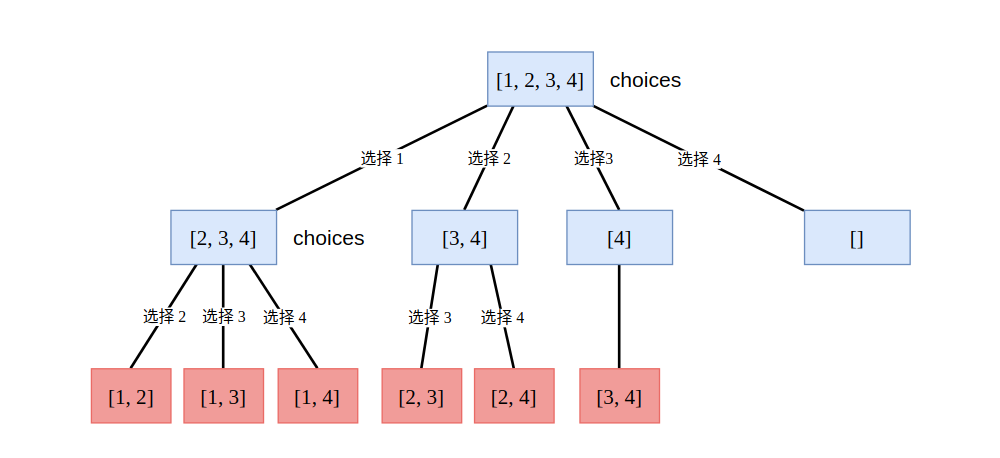``````class Solution {
private:
vector<vector<int>> result;

// 从 start...n 中求解组合问题, selected为已选择路径
void combination(int n, int k, int start, vector<int> &selected) {

if (selected.size() == k) {
result.push_back(selected);
return;
}

for (int i = start; i <= n - (k - selected.size()) + 1; i++) {
selected.push_back(i);                  // 选择
combination(n, k, i + 1, selected);
selected.pop_back();                    // 撤销选择
}
return;
}
public:
vector<vector<int>> combine(int n, int k) {

assert(n > 0 && k > 0 && k <= n);

vector<int> selected;
combination(n , k, 1, selected);

return result;
}
};
``````

### 3. 剪枝

Input: nums = [1,1,2] Output: [[1, 1, 2], [1, 2, 1], [2, 1, 1]]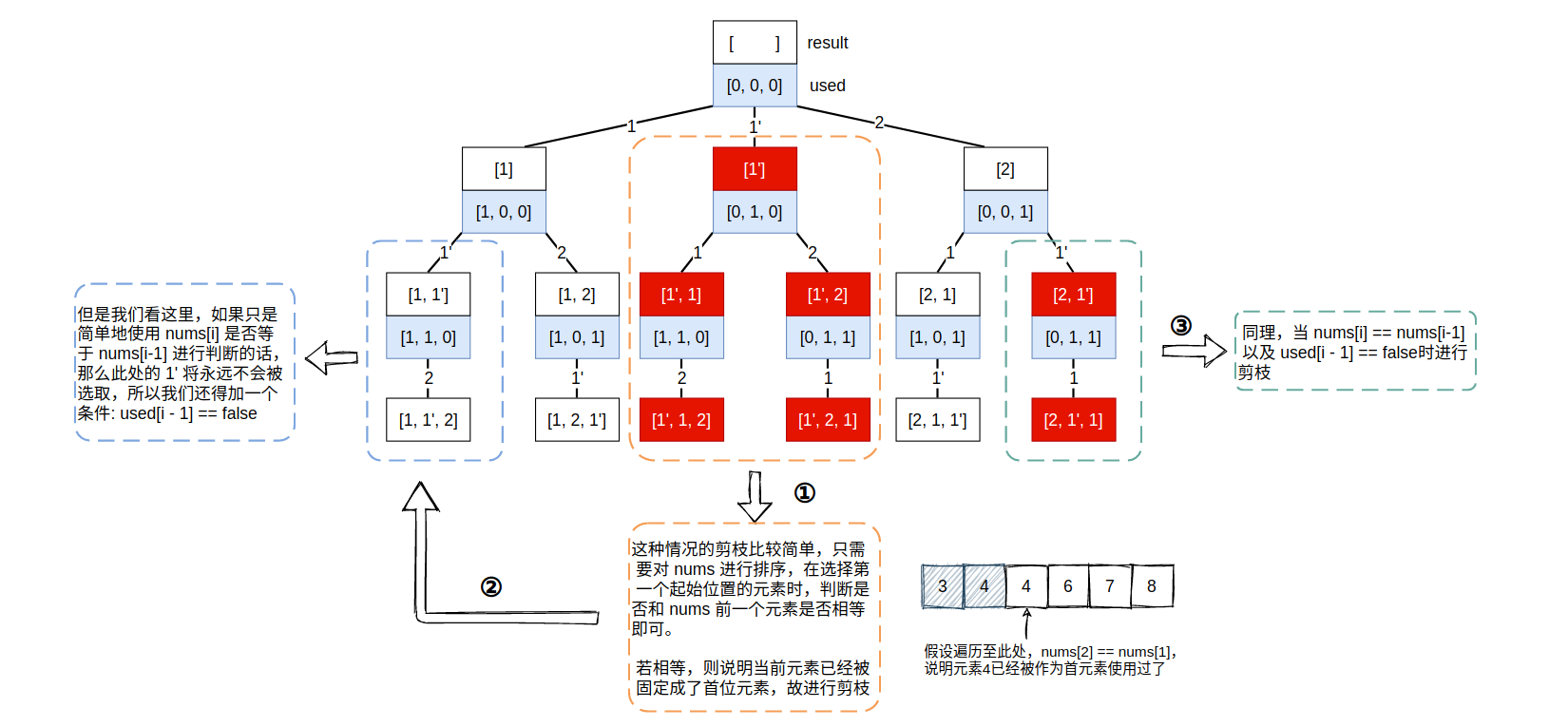https://github.com/SmartKeyerror/Snorlax/blob/master/leetcode/prune/047-Permutations-II.cpp

https://github.com/SmartKeyerror/Snorlax/tree/master/leetcode/prune

### 4. 二维平面中的回溯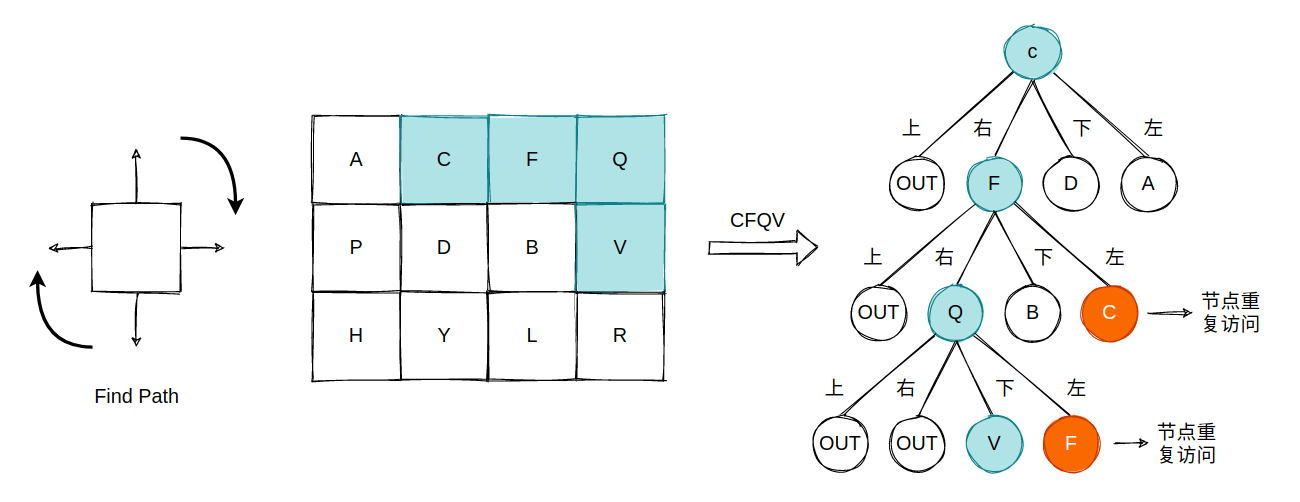https://github.com/SmartKeyerror/Snorlax/blob/master/leetcode/backtracking/079-Word-Search.cpp

### 5. Flood fill

Flood fill 算法又称为漫水填充算法，在扫雷游戏中用于计算需要被清除的区域。200. Number of Islands 该题中我们就可以使用 Flood fill 算法来完成。相较于排列和组合问题有着明显的递归终止条件，Flood fill 算法则需要确保所有选择过的元素的 4 个方向上的元素均被访问并标记过，除非遇到边界。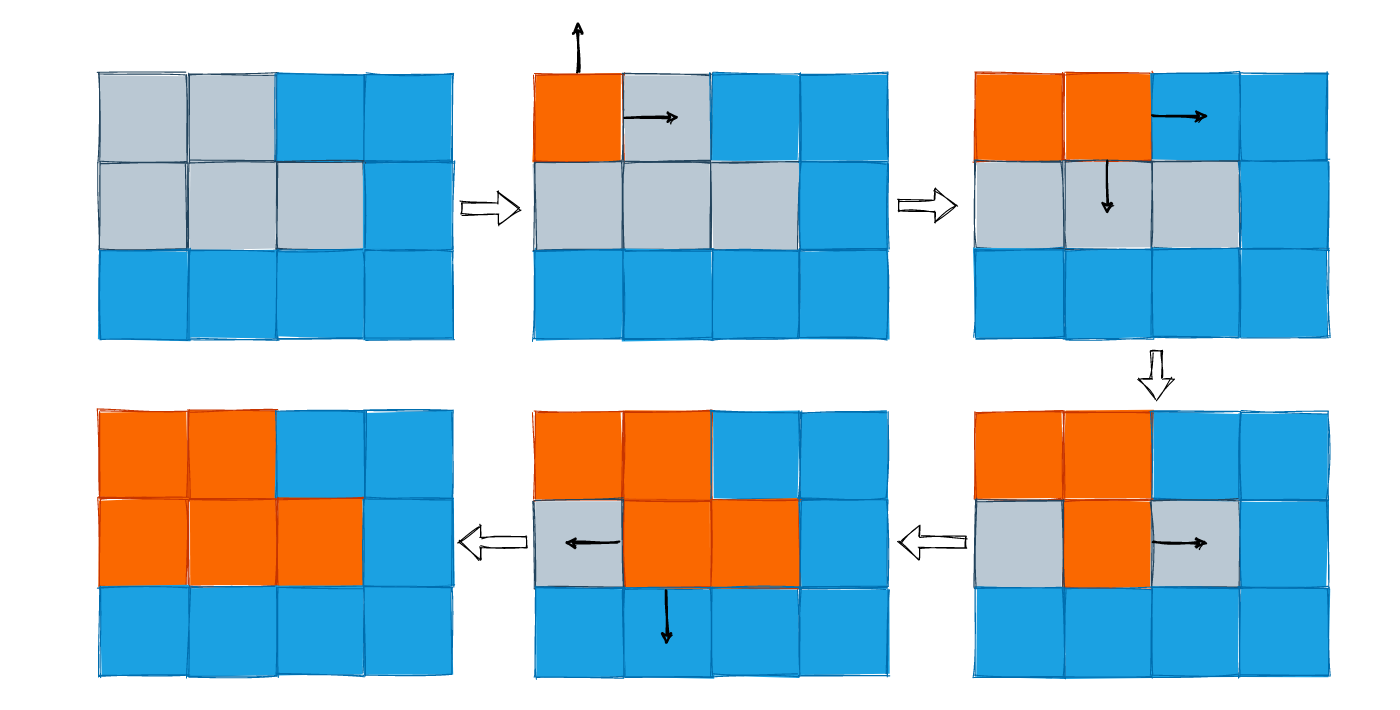https://github.com/SmartKeyerror/Snorlax/blob/master/leetcode/depth-first-search/200-Number-of-Islands.cpp

### 6. 二叉树中的回溯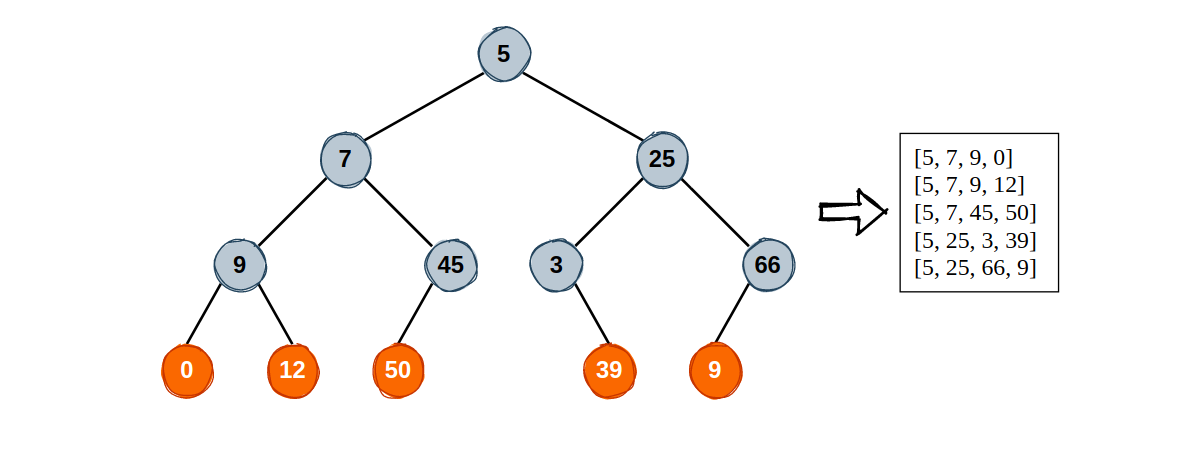``````struct TreeNode {
int val;
TreeNode *left;
TreeNode *right;
};

vector<vector<int>> result;

void treePaths(TreeNode *root, vector<int> &selected) {
if (root == NULL)
return ;

selected.push_back(root->val);

if (root->left == nullptr && root->right == nullptr) {
result.push_back(selected);
selected.pop_back();
return ;
}

treePaths(root->left, selected);
treePaths(root->right, selected);

selected.pop_back();
}
``````

Path Sum IISum Root to Leaf NumbersSmallest String Starting From Leaf 这些问题中都涉及到了二叉树的回溯。

### 7. 小结

leetcode 关于回溯这一话题的全部题目:

https://leetcode.com/tag/backtracking/

https://github.com/SmartKeyerror/Snorlax/tree/master/leetcode/backtracking https://github.com/SmartKeyerror/Snorlax/tree/master/leetcode/prune